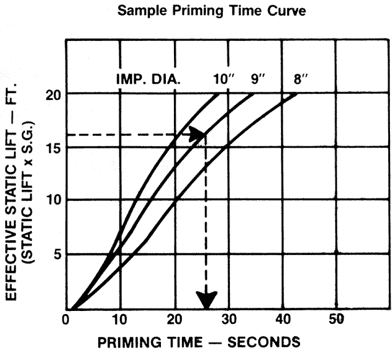### PrimingTimeCalculations

Priming time data for each Model 3796 pump size and speed is displayed on the individual performance curves where priming time is plotted versus effective static lift for maximum, minimum and intermediate Impeller diameters. This data is for suction piping of the same nominal diameter as the pump suction, i.e. 3" piping and 3" pump suction, and must be corrected for suction pipe diameters different from the pump suction and for suction pipe lengths greater than the effective static lift. To calculate the total priming time for a given system:
1. Select the correct size and speed pump from the performance curve for the given rating.
2. Calculate the NPSH Available for the system. The available NPSH must be equal to or greater than the NPSH Required by the selected pump at the rating point.

NPSHA = P-(Ls + Vp + hf)
where:
P = Pressure on surface of liquid in feet absolute

L s= Maximum static lift in feet from free surface of the liquid to the centerline of the impeller.
V p =Vapor pressure of the liquid at maximum pumping temperature in feet absolute.
h f = Suction pipe friction loss in feet at the required capacity. 3. Determine the effective static lift.
L es = Ls x Sp. Gr. where: L es = Effective static lift in feet.
Ls = Maximum static lift in feet from free surface of the liquid to the centerline of the pump suction, or the highest point in the suction piping, whichever is greater.
Sp. Gr. = Specific gravity of the liquid. 4. Enter the priming time curve at the effective static lift calculated in Step 3. Proceed across to the impeller diameter selected for the specified rating and then downward to the bottom coordinate to determine the priming time (PTLes ) to achieve the given lift.
5. insert the priming time from Step 4 into the following formula to calculate the total system priming time:where:
PT T = Total system priming time.
PT LES = Priming time in seconds for the effective static lift (Step 4.)
SPL = Total suction pipe length above the free surface of the liquid in feet.
L es = Effective static lift.
D p = Nominal pipe diameter.
D s = Nominal pump suction diameter.